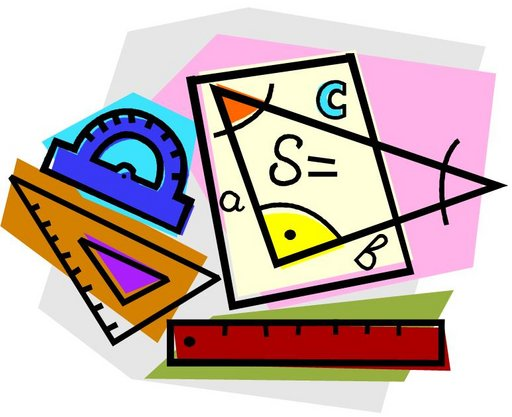# Lines, Angles and Shapes

##BIG IDEAS:

(taken from “Big Ideas by Dr. Small”):

1. Some attributes of shapes are quantitative, others are qualitative.
2. What makes shapes alike and different can be determined by geometric properties. For example, shapes have sides that are parallel, perpendicular or neither; they have lines of symmetry, rotational symmetry, or neither.
3. Many geometric properties and attributes of shapes are related to measurement, for example, how far apart two parallel lines are or how the side lengths of a shape compare.

## STUDENT LEARNING GOALS:

GOAL #1: I can construct parallel, intersecting and perpendicular lines on paper and computer applications.

GOAL #2: I can construct angle and perpendicular bisectors on paper and computer applications.

GOAL #3: I can construct triangles with given conditions and explore the properties of triangles.

GOAL #4: I can sort, classifying and construct a variety of different quadrilaterals.

CURRICULUM EXPECTATIONS:

• Construct related lines, and classify triangles, quadrilaterals, and prisms;
• Construct related lines (i.e., parallel; perpendicular; intersecting at 30º, 45º, and 60º), using angle properties and a variety of tools (e.g., compass and straight edge, protractor, dynamic geometry software) and strategies (e.g., paper folding);
• Sort and classify triangles and quadrilaterals by geometric properties related to symmetry, angles, and sides, through investigation using a variety of tools (e.g., geoboard, dynamic geometry software) and strategies (e.g., using charts, using Venn diagrams);
• Construct angle bisectors and perpendicular bisectors, using a variety of tools (e.g., Mira, dynamic geometry software, compass) and strategies (e.g., paper folding), and represent equal angles and equal lengths using mathematical notation;